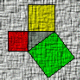# Displaying variables and characters in an entmake dimension## Recommended PostsHello! I just stared working in AutoLISP a few days ago, and I've come across a problem. I need to create a dimension line where the dimension value is dependent on an input value. So if the input is y, then the text on the dimension line should equal 4.5*(y+1) inches, and it also needs to have a character width of 0.75. Currently, it just displays 4 1/2". As I understand it, this would be a real number, meaning I can't concatenate it with the inches symbol. Any help would be greatly appreciated!```(defun c:retan (/ p1 p2 p3 p4 x y i j)(setvar "osmode" 0)

(setq p1 (getpoint "\nfirst corner of rectangle: "))
(setq x (getint "\nEnter Horizontal Count: "))
(setq y (getint "\nEnter Vertical Count: "))

(setq p3 (list (+ (+ 1.625 (* 0.8125 (- x 1))) (car p1))(+ (+ 1.625 (* 0.8125 (- y 1))) (cadr p1))))
(setq p2 (list (car p1)(cadr p3)))
(setq p4 (list (car p3)(cadr p1)))
(command "pline" p1 p2 p3 p4 "c") ;c closes the rectangle's fourth side

(entmakex (list
(cons 0 "DIMENSION")
(cons 100 "AcDbEntity")
(cons 8 "E-DIMS")                                                       ;; 8 Layer
(cons 100 "AcDbDimension")
(cons 10 (list (- (car P1) 0.7) (cadr P2) 0))   ;; 10 Arrow Node
(cons 11 (list (- (car P1) 0.9) (/ (+ (cadr P2)(cadr P1)) 2) 0))        ;; 11 Text Position
(cons 70 160)
(cons 1 "{\\W0.75;4 1/2\"}")                                            ;; 1  Contents of Dimension Textbox
(cons 71 5)                                                             ;; 71 Text Alignment (5=centered)
(cons 42 0.8125)
(cons 53 1.5708)                                                        ;; 53 Text Rotation
(cons 3 "REW-1_.125txt")
(cons 100 "AcDbAlignedDimension")
(cons 13 P1)                                                            ;; 13 point on line
(cons 14 P2)                                                            ;; 14 point on line
(cons 50 1.5708)                                                        ;; 50 Angle (radians) 1.5708
(cons 100 "AcDbRotatedDimension")))

(setvar "osmode" 16383)(princ)
)```

##### Share on other sites```(cons 1 (strcat  "{\\W0.75;" (rtos (* 4.5 (1+ y)) 4 2) "}"))
```

ymg

##### Share on other sitesThanks ymg, that did the trick!

## Join the conversation

You can post now and register later. If you have an account, sign in now to post with your account.
Note: Your post will require moderator approval before it will be visible.×   Pasted as rich text.   Restore formatting

Only 75 emoji are allowed.